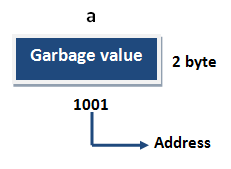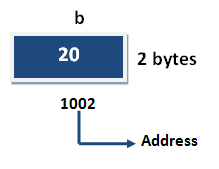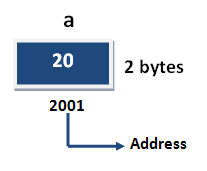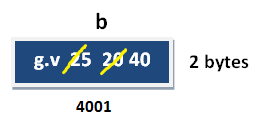# Variable in C++

## What are Variables

Variable are used in C++, where we need storage for any value, which will change in program. Variable can be declared in multiple ways each with different memory requirements and functioning. Variable is the name of memory location allocated by the compiler depending upon the datatype of the variable.

## Basic types of Variables

Each variable while declaration must be given a datatype, on which the memory assigned to the variable depends. Following are the basic types of variables,

 `bool` For variable to store boolean values( True or False ) `char` For variables to store character types. `int` for variable with integral values `float` and `double` are also types for variables with large and floating point values

## Variable declarations

This is the process of allocating sufficient memory space for the data in term of variable.

## Syntax

Datatype variable_name; int a;If no input values are assigned by the user than system will gives a default value called garbage value.

## Garbage value

Garbage value can be any value given by system and that is no way related to correct programs.
It is a disadvantage and it can overcome using variable initialization.

## Variable initialization

It is the process of allocating sufficient memory space with user defined values.

## Syntax

```Datatype nariable_name=value;
```

## Example

```int  b = 30;
```## Variable assignment

It is a process of assigning a value to a variable.

## Syntax

Variable_Name = value

## Example

```int  a= 20;
int b;
```## Example

```b = 25; // --> direct assigned variable
b = a;  // --> assigned value in term of variable
b = a+15;  // --> assigned value as term of expression
```## Rule to Declare Variable in C++

To Declare any variable in C++ you need to follow rules and regulation of C++ Language, which is given below;

• Every variable name should start with alphabets or underscore (_).
• No spaces are allowed in variable declaration.
• Except underscore (_) no special symbol are allowed in the middle of the variable declaration.
• Maximum length of variable is 8 characters depend on compiler and operation system.
• Every variable name always should exist in the left hand side of assignment operator.
• No keyword should access variable name.

Note: In a c program variable name always can be used to identify the input or output data.

## Scope of Variable in C++

In C++ language, a variable can be either of global or local scope.

## Global variable

Global variables are defined outside of all the functions, generally on top of the program. The global variables will hold their value throughout the life-time of your program.

## Local variable

A local variable is declared within the body of a function or a block. Local variable only use within the function or block where it is declare.

## Example

```#include<iostream.h>
#include<conio.h>

int a;   // global variable
void main()
{
int b;    // local variable
a=10, b=20;
cout<<"Value of a: "<<a;
cout<<"Value of b: "<<b;
getch();
}```

## Output

```Value of a: 10
Value of b: 20```